Скачать презентацию Inferring Mixtures of Markov Chains Tuğkan Batu Sudipto

dd25cec19defcc2632b0f2483de82326.ppt

• Количество слайдов: 29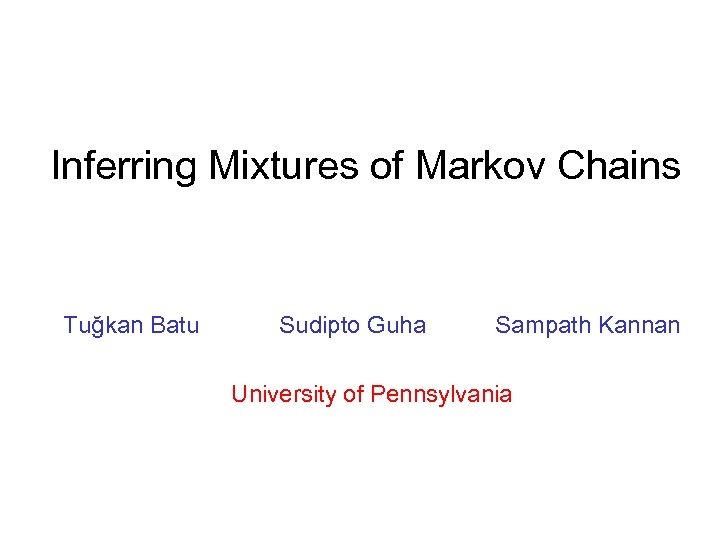Inferring Mixtures of Markov Chains Tuğkan Batu Sudipto Guha Sampath Kannan University of Pennsylvania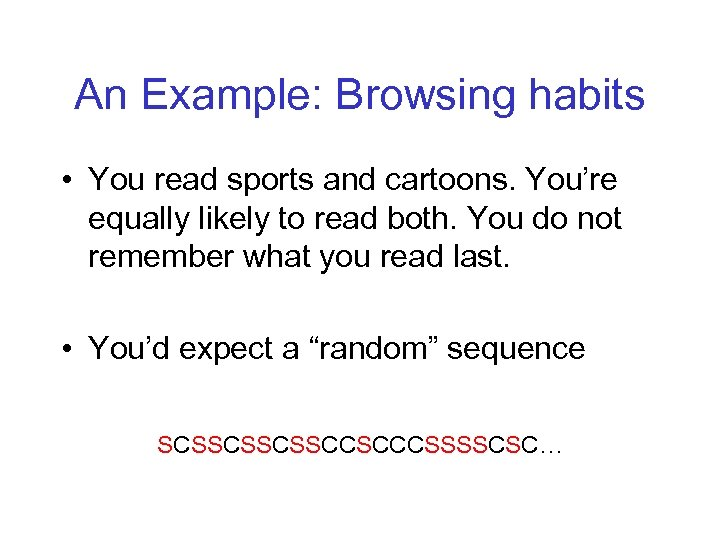An Example: Browsing habits • You read sports and cartoons. You’re equally likely to read both. You do not remember what you read last. • You’d expect a “random” sequence SCSSCSSCSSCCSCCCSSSSCSC…Suppose there were two • I like health and entertainment • I always read entertainment first and then read health page. • The sequence would be EHEHEHEH…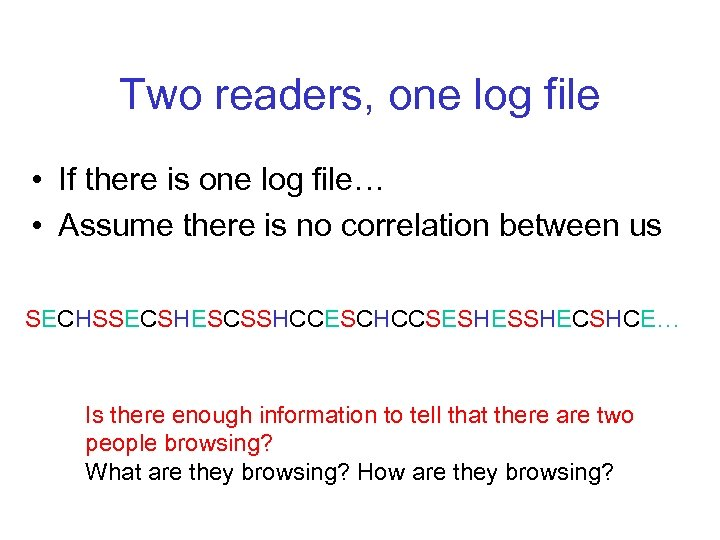Two readers, one log file • If there is one log file… • Assume there is no correlation between us SECHSSECSHESCSSHCCESCHCCSESHESSHECSHCE… Is there enough information to tell that there are two people browsing? What are they browsing? How are they browsing?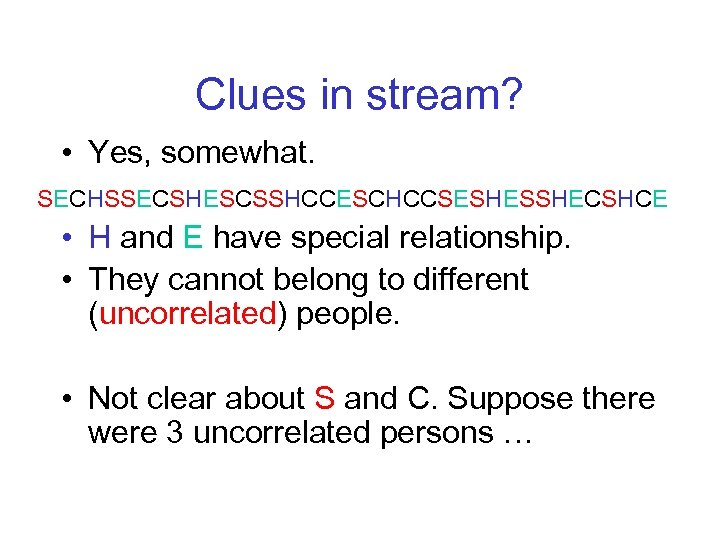Clues in stream? • Yes, somewhat. SECHSSECSHESCSSHCCESCHCCSESHESSHECSHCE • H and E have special relationship. • They cannot belong to different (uncorrelated) people. • Not clear about S and C. Suppose there were 3 uncorrelated persons …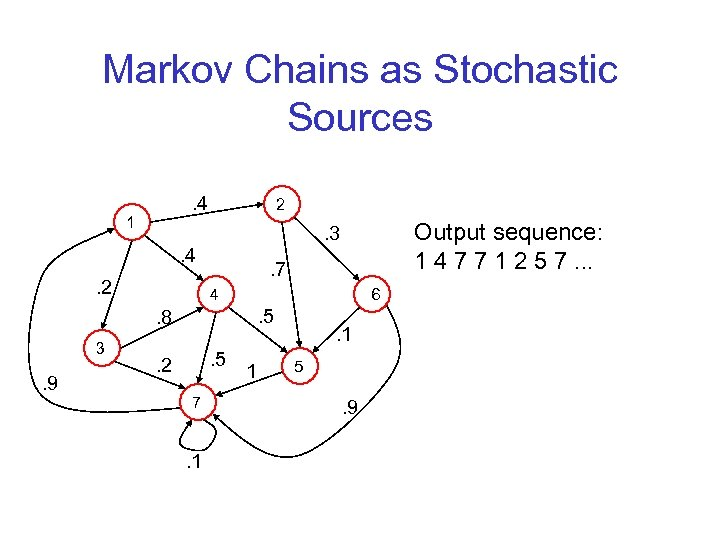Markov Chains as Stochastic Sources. 4 1 2 . 4. 2 3 . 9 . 7 4 . 8 . 5 . 2 7 . 1 Output sequence: 1 4 7 7 1 2 5 7. . 3 6 . 5 1 . 1 5 . 9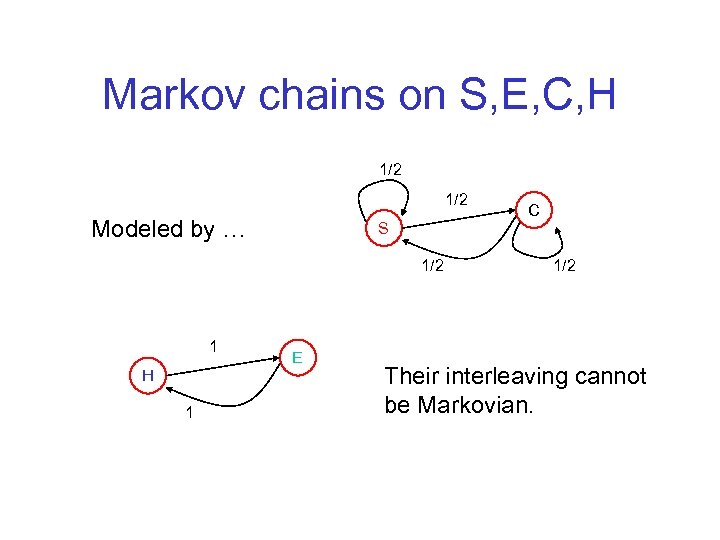Markov chains on S, E, C, H 1/2 Modeled by … S 1/2 1 H 1 E C 1/2 Their interleaving cannot be Markovian.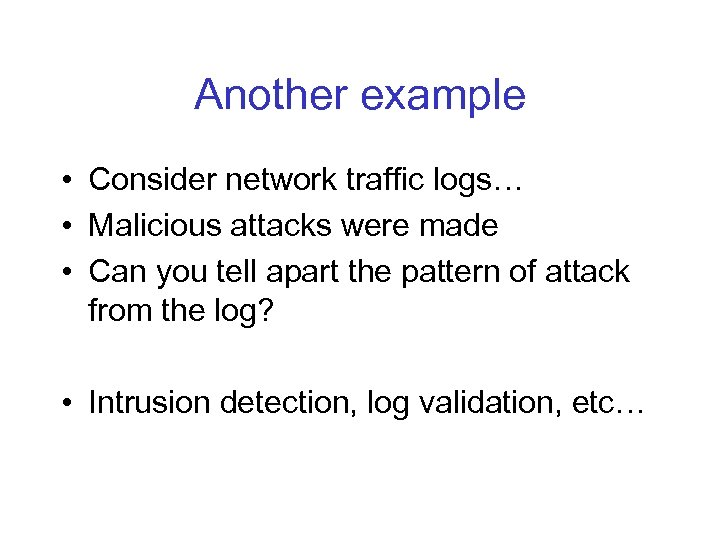Another example • Consider network traffic logs… • Malicious attacks were made • Can you tell apart the pattern of attack from the log? • Intrusion detection, log validation, etc…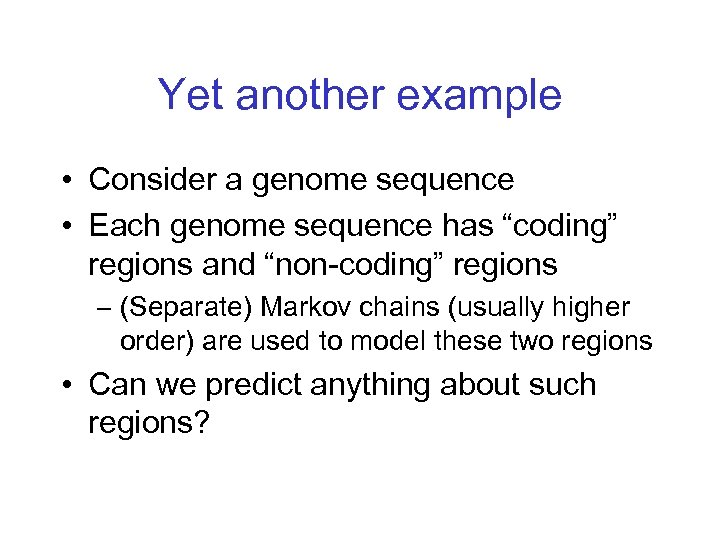Yet another example • Consider a genome sequence • Each genome sequence has “coding” regions and “non-coding” regions – (Separate) Markov chains (usually higher order) are used to model these two regions • Can we predict anything about such regions?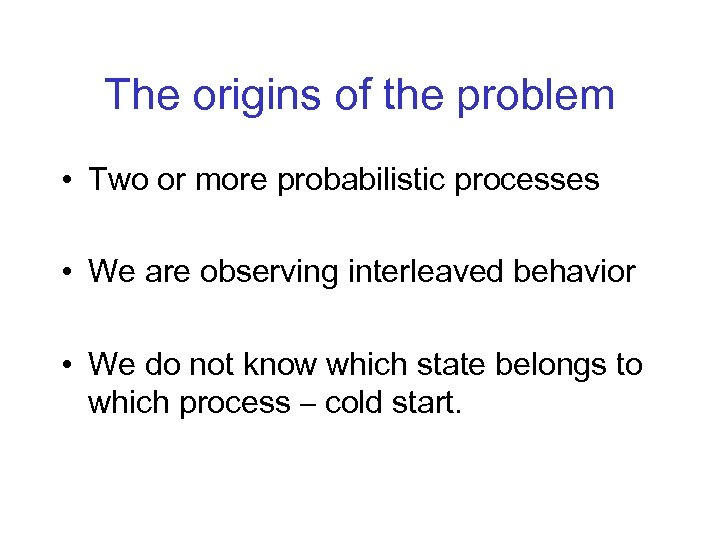The origins of the problem • Two or more probabilistic processes • We are observing interleaved behavior • We do not know which state belongs to which process – cold start.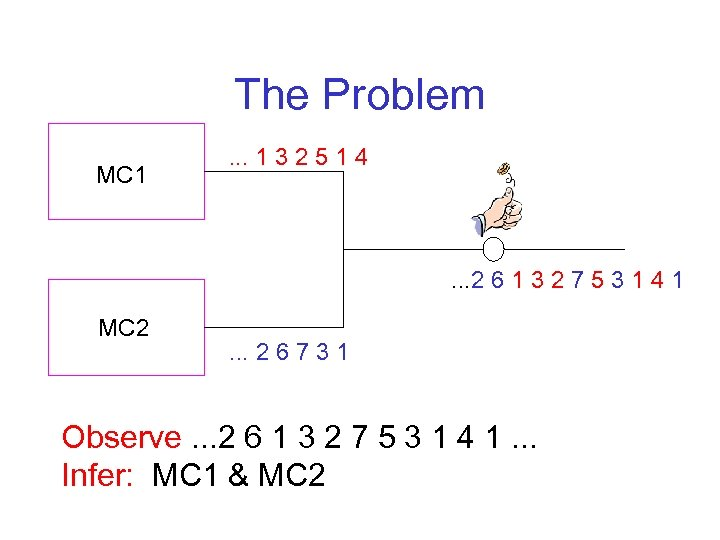The Problem MC 1 . . . 1 3 2 5 1 4 . . . 2 6 1 3 2 7 5 3 1 4 1 MC 2 . . . 2 6 7 3 1 Observe. . . 2 6 1 3 2 7 5 3 1 4 1. . . Infer: MC 1 & MC 2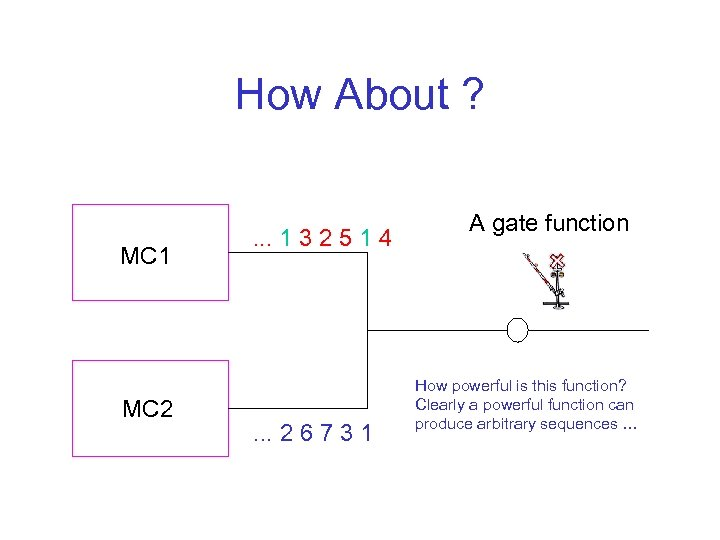How About ? MC 1 MC 2 . . . 1 3 2 5 1 4 . . . 2 6 7 3 1 A gate function How powerful is this function? Clearly a powerful function can produce arbitrary sequences …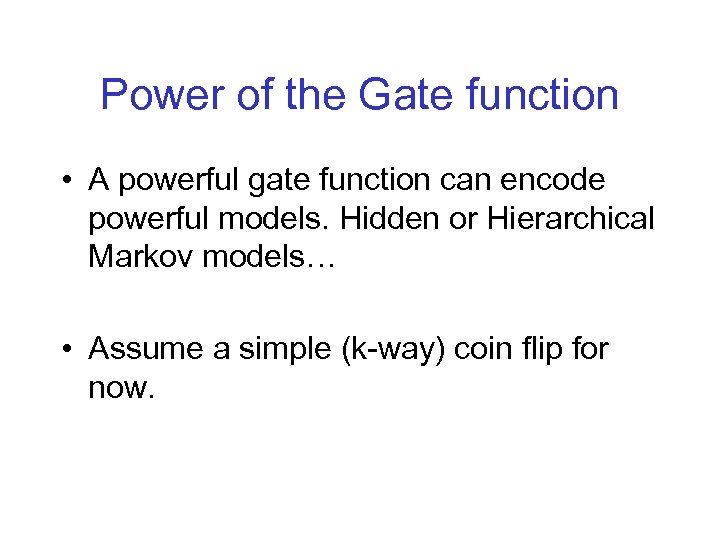Power of the Gate function • A powerful gate function can encode powerful models. Hidden or Hierarchical Markov models… • Assume a simple (k-way) coin flip for now.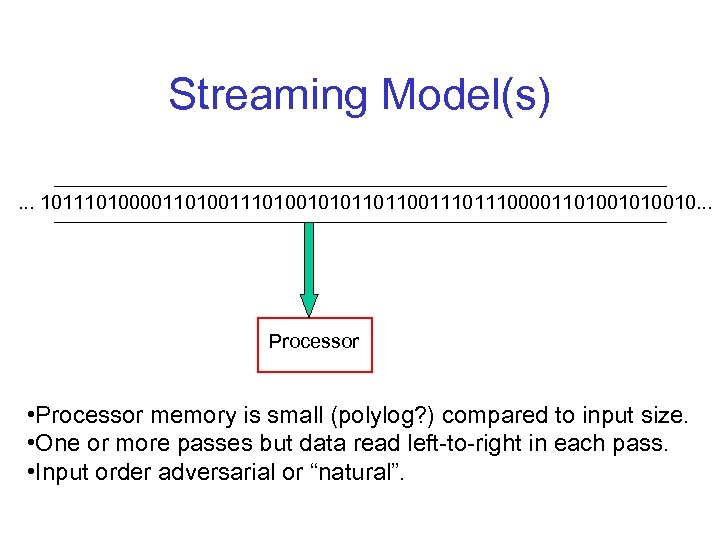Streaming Model(s). . . 1011101000011010010101101100111000011010010. . . Processor • Processor memory is small (polylog? ) compared to input size. • One or more passes but data read left-to-right in each pass. • Input order adversarial or “natural”.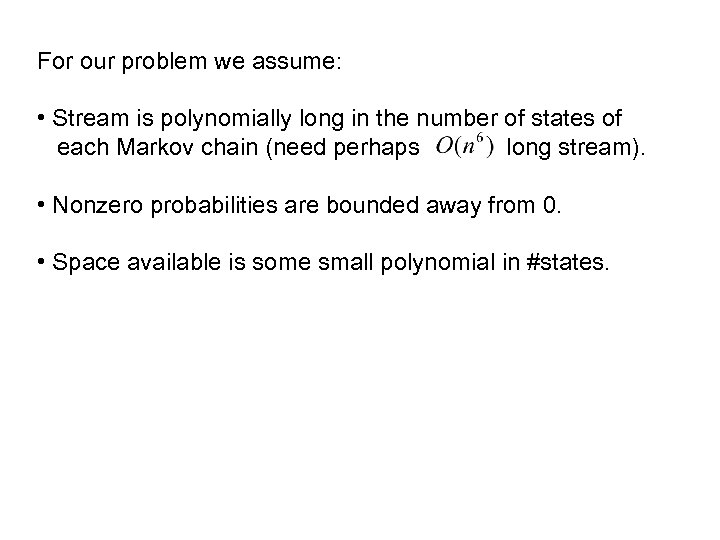For our problem we assume: • Stream is polynomially long in the number of states of each Markov chain (need perhaps long stream). • Nonzero probabilities are bounded away from 0. • Space available is some small polynomial in #states.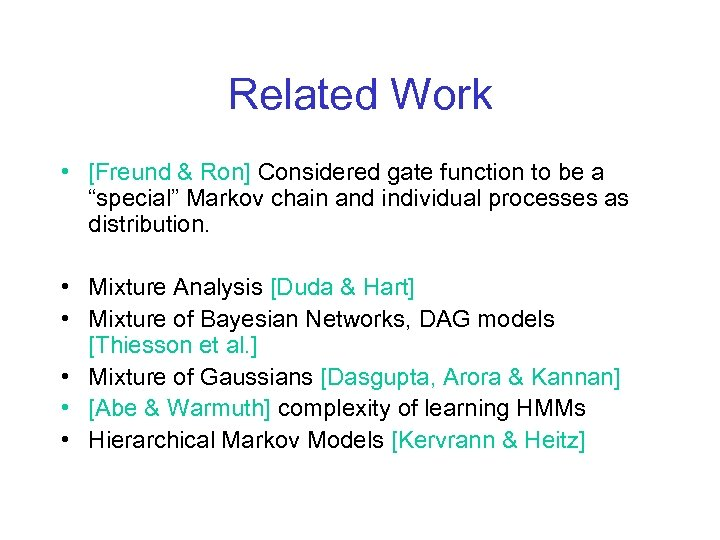Related Work • [Freund & Ron] Considered gate function to be a “special” Markov chain and individual processes as distribution. • Mixture Analysis [Duda & Hart] • Mixture of Bayesian Networks, DAG models [Thiesson et al. ] • Mixture of Gaussians [Dasgupta, Arora & Kannan] • [Abe & Warmuth] complexity of learning HMMs • Hierarchical Markov Models [Kervrann & Heitz]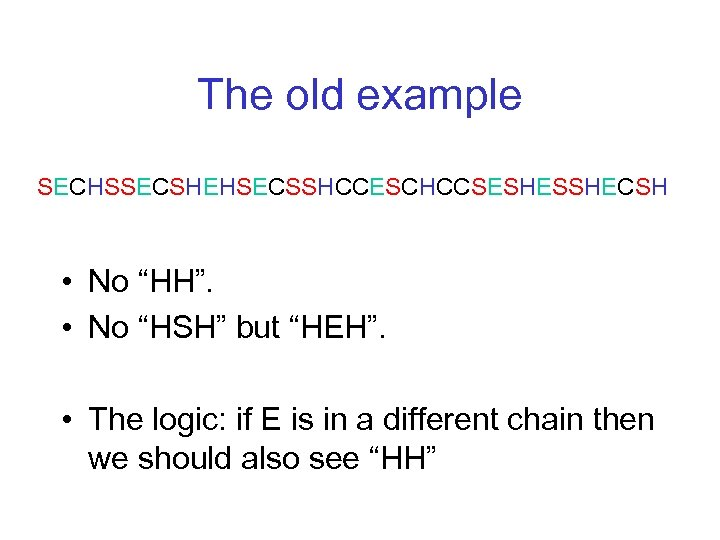The old example SECHSSECSHEHSECSSHCCESCHCCSESHESSHECSH • No “HH”. • No “HSH” but “HEH”. • The logic: if E is in a different chain then we should also see “HH”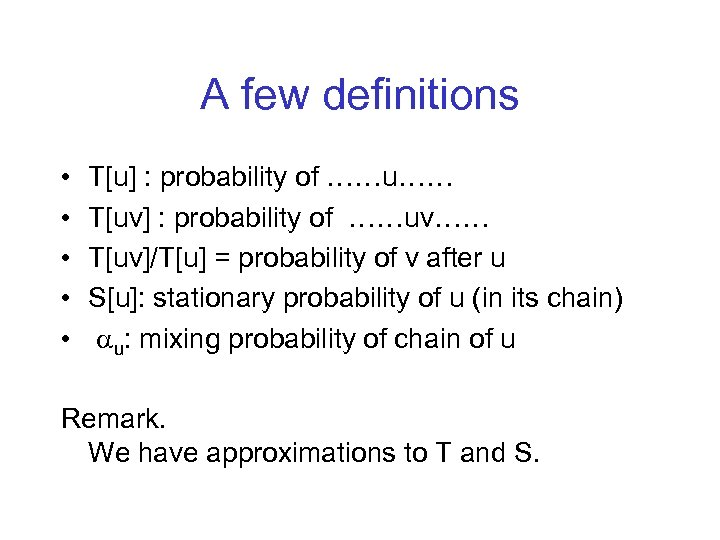A few definitions • • • T[u] : probability of ……u…… T[uv] : probability of ……uv…… T[uv]/T[u] = probability of v after u S[u]: stationary probability of u (in its chain) au: mixing probability of chain of u Remark. We have approximations to T and S.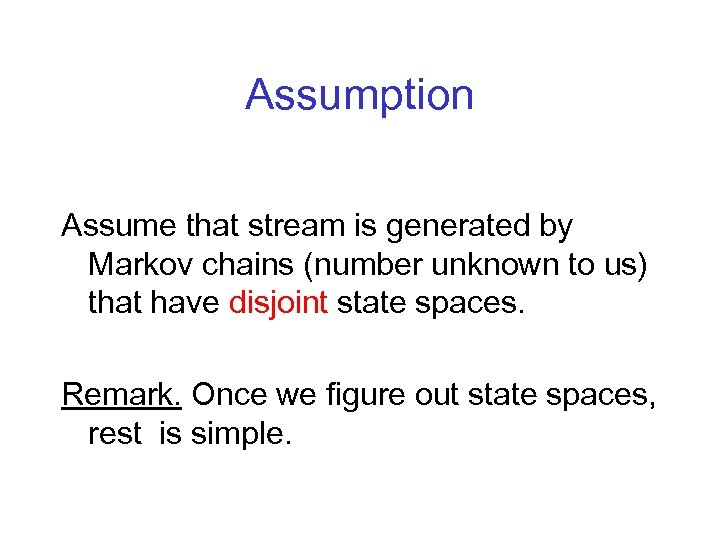Assumption Assume that stream is generated by Markov chains (number unknown to us) that have disjoint state spaces. Remark. Once we figure out state spaces, rest is simple.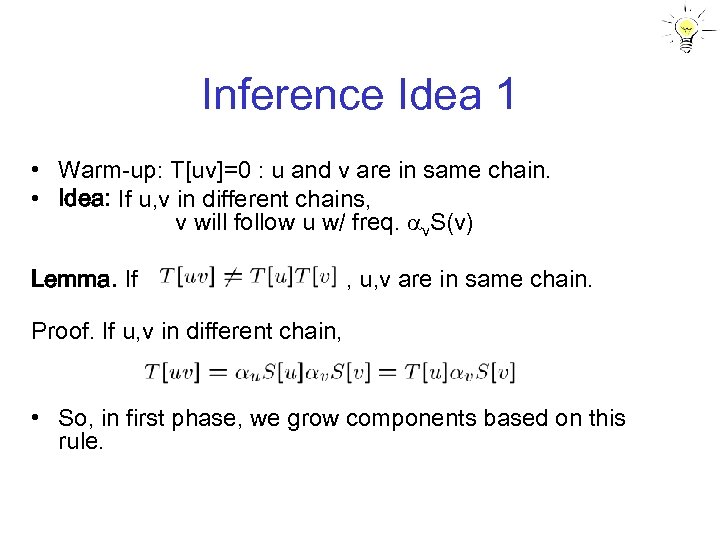Inference Idea 1 • Warm-up: T[uv]=0 : u and v are in same chain. • Idea: If u, v in different chains, v will follow u w/ freq. av. S(v) Lemma. If , u, v are in same chain. Proof. If u, v in different chain, • So, in first phase, we grow components based on this rule.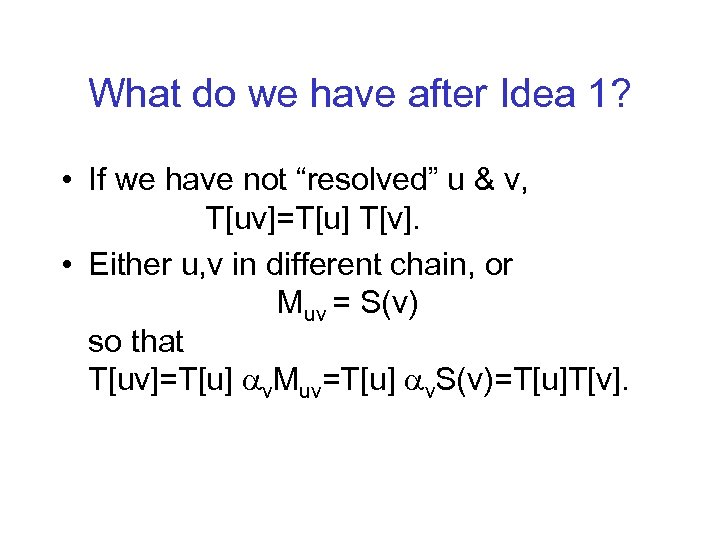What do we have after Idea 1? • If we have not “resolved” u & v, T[uv]=T[u] T[v]. • Either u, v in different chain, or Muv = S(v) so that T[uv]=T[u] av. Muv=T[u] av. S(v)=T[u]T[v].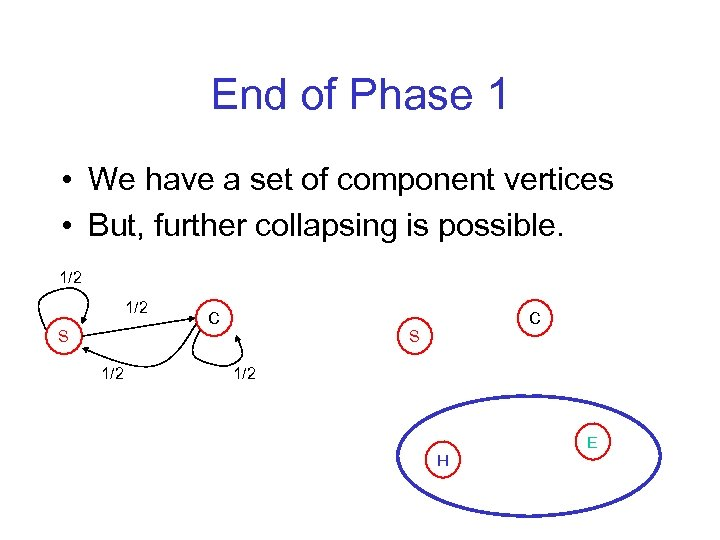End of Phase 1 • We have a set of component vertices • But, further collapsing is possible. 1/2 S 1/2 C C S 1/2 H E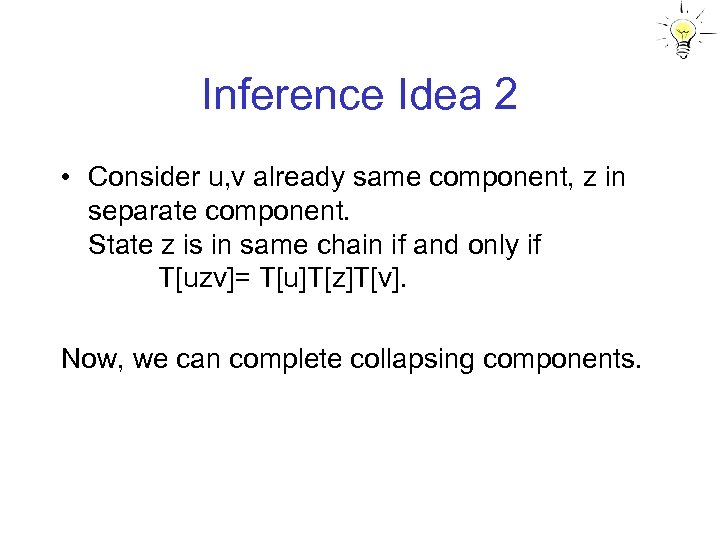Inference Idea 2 • Consider u, v already same component, z in separate component. State z is in same chain if and only if T[uzv]= T[u]T[z]T[v]. Now, we can complete collapsing components.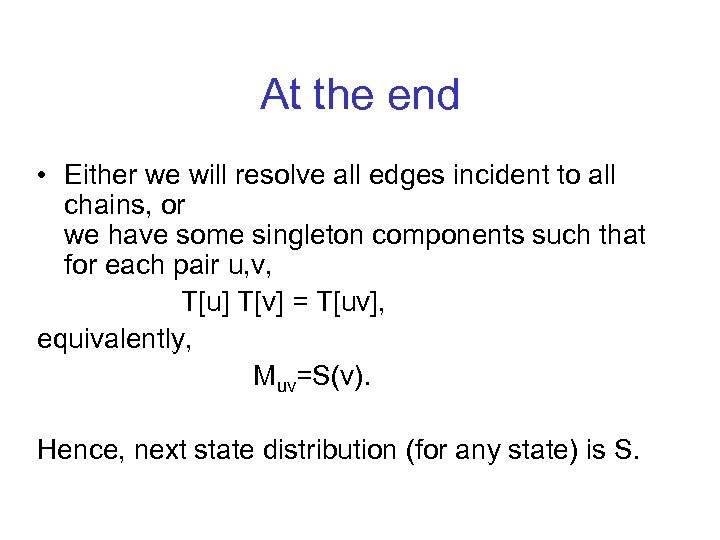At the end • Either we will resolve all edges incident to all chains, or we have some singleton components such that for each pair u, v, T[u] T[v] = T[uv], equivalently, Muv=S(v). Hence, next state distribution (for any state) is S.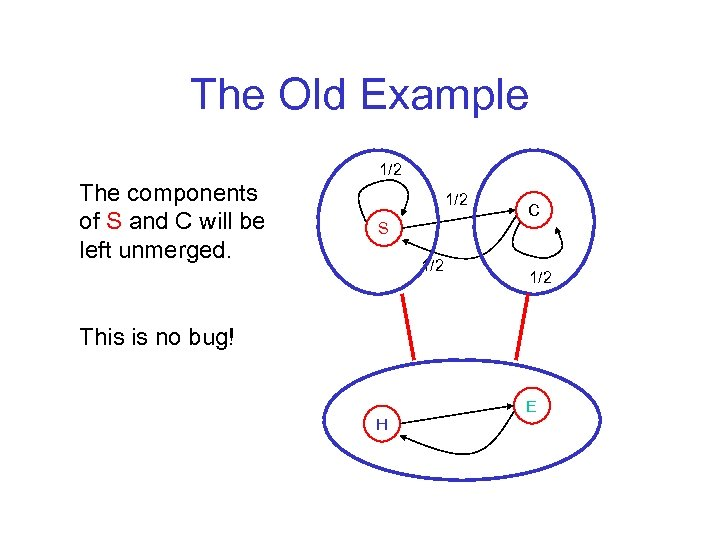The Old Example The components of S and C will be left unmerged. 1/2 S 1/2 C 1/2 This is no bug! H E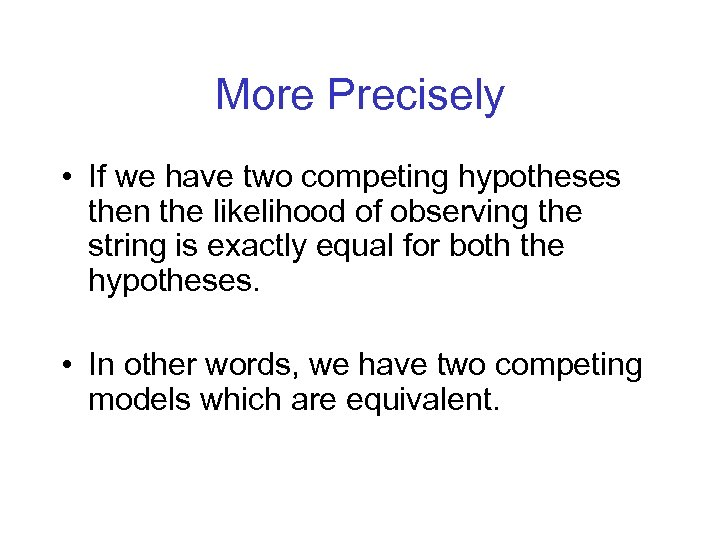More Precisely • If we have two competing hypotheses then the likelihood of observing the string is exactly equal for both the hypotheses. • In other words, we have two competing models which are equivalent.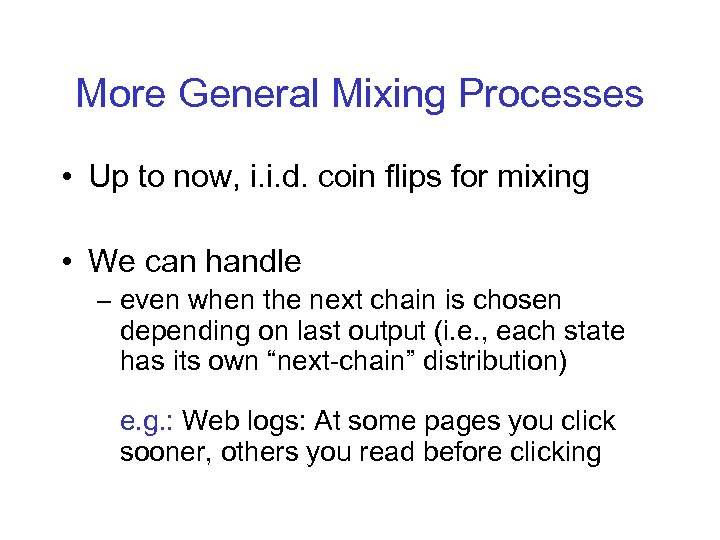More General Mixing Processes • Up to now, i. i. d. coin flips for mixing • We can handle – even when the next chain is chosen depending on last output (i. e. , each state has its own “next-chain” distribution) e. g. : Web logs: At some pages you click sooner, others you read before clicking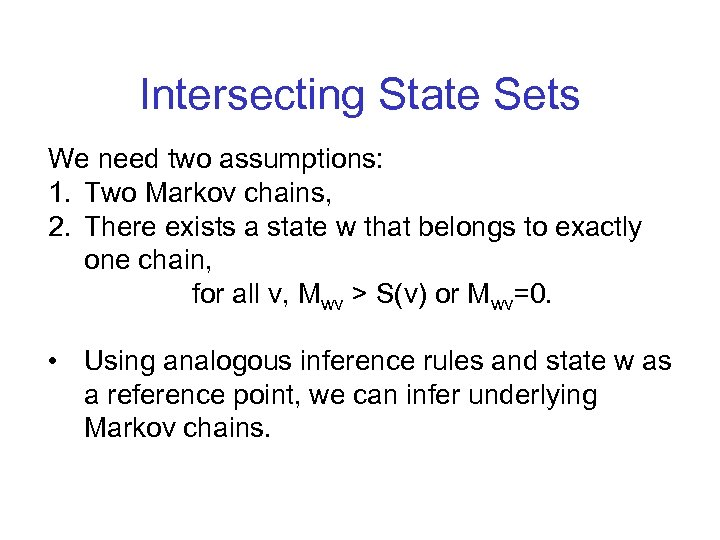Intersecting State Sets We need two assumptions: 1. Two Markov chains, 2. There exists a state w that belongs to exactly one chain, for all v, Mwv > S(v) or Mwv=0. • Using analogous inference rules and state w as a reference point, we can infer underlying Markov chains.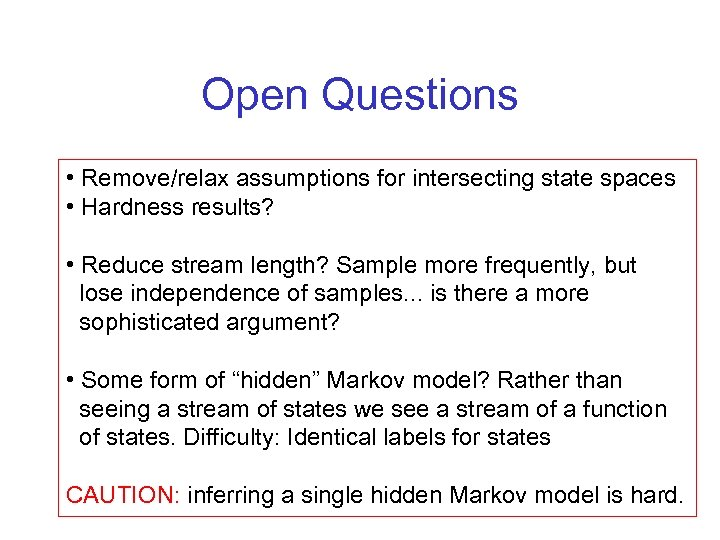Open Questions • Remove/relax assumptions for intersecting state spaces • Hardness results? • Reduce stream length? Sample more frequently, but lose independence of samples. . . is there a more sophisticated argument? • Some form of “hidden” Markov model? Rather than seeing a stream of states we see a stream of a function of states. Difficulty: Identical labels for states CAUTION: inferring a single hidden Markov model is hard.